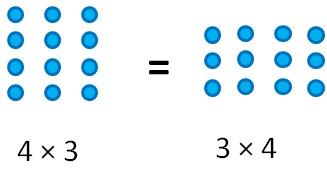Properties of Rational Number

Chapter 1 Class 8 Rational Numbers
Concept wise
 Operation Commutative Closed or not Addition a  + b = b + a True Subtraction a  − b = b − a False Multiplication a × b = b × a True Division a/b = b/a FalseSo commutativity is always possible for addition &

multiplication, but not for subtraction & division.

For Rational Numbers

Let us take two rational numbers 1/2 & 3/2

 Operation Number Remark Addition a + b = b + a Take a = 1/2 & b = 3/2   L.H.S a + b = 1/2+3/2 = (1 +  3)/2 = 4/2 = 2   R.H.S b + a = 3/2 + 1/2  = (3 +  1)/2 = 4/2 = 2   ∴ a + b = b + a Since a + b = b + a, ∴ Addition is commutative. Subtraction a − b = b − a Take a = 1/2 & b = 3/2   L.H.S a − b = 1/2-3/2 = (1 -  3)/2 = (-2)/2 = −1   R.H.S b – a = 3/2- 1/2  = (3 -  1)/2 = 2/2 = 1 ∴ a − b ≠ b – a Since a − b ≠ b − a, ∴ Subtraction is not commutative . Multiplication a ×  b =  b × a Take a = 1/2, b = 3/2   L.H.S a × b = 1/2×  3/2 = (1 × 3)/(2 × 2) = 3/4   R.H.S b × a = 3/2×1/2  = (3  ×  1)/(2  ×  2) = 3/4   ∴ a × b = b × a Since, a × b = b × a ∴ Multiplication is commutative. Division a/b=b/a Take a = 1/2 , b = 3/2   L.H.S  a/b = (1/2  )/(3/2) = 1/2×2/3 = 1/3   R.H.S  b/a = (3/2  )/(1/2) = 3/2×2/1 = 3   ∴ a/b≠b/a Since a/b≠b/a  ∴ Division is not commutative .

#### To summarize

 Numbers Commutative for Addition Subtraction Multiplication Division Natural numbers Yes No Yes No Whole numbers Yes No Yes No Integers Yes No Yes No Rational Numbers Yes No Yes No

So commutativity is always possible for addition & multiplication,

but not for subtraction & division.

Learn in your speed, with individual attention - Teachoo Maths 1-on-1 Class